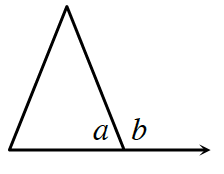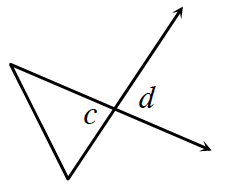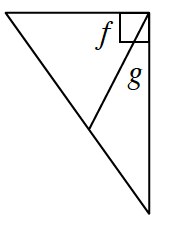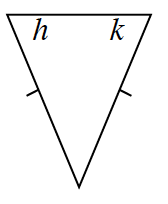### Home > INT2 > Chapter 1 > Lesson 1.3.1 > Problem1-75

1-75.

Examine the diagrams below. For each pair of angles marked on the diagram, quickly decide what angle pair relationship(s) the angles have. You may list more than one relationship for each pair of angles.

1.linear pair or straight angle pair, supplementary

1.1.These angles add to $90$ degrees.

1.These angles are equal in measure.